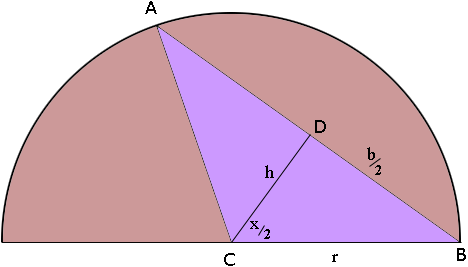Name: Howard Who is asking: Other Level of the question: Secondary Question: I thought of the following problem which is similar but much simpler than the tethered goat problem: What is the angle(it is more illustrative in degrees)of arc of a unit circle so that the area between the chord it subtends and the arc length is equal to the area of the triangle with opposite side the subtended chord. (Sorry, it would have been easier to state the problem with a diagram).[I don't need the solution, but thought it would be fun for others] Howard, Below is our diagram of your problem.The problem is to find the measure of the angle ACB so that the area of the triangle ACB is equal to the area of the region of the sector ACB that is outside the triangle. To say it in another way, find the measure of the angle ACB if the area of the triangle ACB is half the area of the sector ACB. Let x be the measure of the angle ACB in radians and r be the radius of the circle, then the area of the sector ACB is AS = 1/2 r2 x Let D be the midpoint of AB, b = |AB| and h = |CD| then the area of the triangle ACB is AT = 1/2 b h Using the facts that h = r cos(  x/2 ), b = 2r sin( x/2 ) and sin(x) = 2 sin( x/2 ) cos( x/2 ) we get AT = 1/2 r2 sin(x). But AS = 2 AT and hence 2 sin(x) = x Solving this equation is not straightforward. One technique is to use Newton's method to approximate a solution. There is an outline of Newton's method in the answer to a previous problem. Stephen and Penny